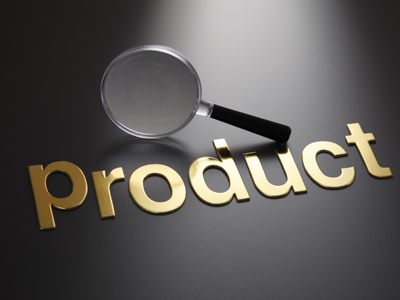In maths, a product is what you get after you multiply two or more numbers.

# Following Instructions 2

Welcome to the second of our Following Instructions quizzes. If you have already played the first, then you will know that the instructions you are asked to follow will involve figures and are a test of how well you know your mathematical terms.

Here are some terms you will need to know:

• Sum of – the result of an addition
• Product – the result of a multiplication
• Quotient – the result of a division

There are a couple more you may need to learn, but I’ll explain those in the next quiz.

Take your time and read each question carefully before you choose your answer. You don't want to make any silly mistakes now, do you?. Good luck!

Example:
Multiply the first figure by the sum of the last two:
8, 5, 4

The sum of the last two digits is 9 (5 + 4 = 9). The answer is 9 x 8 = 72
1.
Multiply the second figure by the sum of the first and last:
22, 4, 3
100
154
75
125
The sum of the first and last figures is 25 (22 + 3 = 25). The answer is 4 x 25 = 100
2.
Multiply the first figure by the sum of the last two:
9, 13, 2
234
34
135
35
The sum of the last two digits is 15 (13 + 2 = 15). The answer is 9 x 15 = 135
3.
Add the last figure to the product of the first two:
4, 5, 17
37
26
45
19
The product of the first two figures is 20 (4 x 5 = 20). The answer is 17 + 20 = 37
4.
Multiply the last figure by the sum of the first two:
7, 5, 8
43
96
280
88
The sum of the first two figures is 12 (7 + 5 = 12). The answer is 8 x 12 = 96
5.
Subtract the first figure from the product of the last two:
32, 10, 5
-17
17
18
28
The product of the last two figures is 50 (10 x 5 = 50). The answer is 50 - 32 = 18
6.
Subtract the third figure from the quotient of the first two (the first figure is the dividend and the second is the divisor):
39, 3, 4
8
10
7
9
The quotient of the first two figures is 13, if 39 is the dividend and 3 the divisor (39 ÷ 3 = 13). The answer is 13 - 4 = 9
7.
Subtract the first figure from the quotient of the last two (the last figure is the dividend and the middle one is the divisor):
7, 4, 64
1
-3
3
9
The quotient of the last two figures is 16, if 64 is the dividend and 4 the divisor (64 ÷ 4 = 16). The answer is 16 - 7 = 9
8.
Add the first figure to the quotient of the last two (the middle figure is the dividend and the last is the divisor):
23, 49, 7
65
79
30
20
The quotient of the last two figures is 7, if 49 is the dividend and 7 the divisor (49 ÷ 7 = 7). The answer is 7 + 23 = 30
9.
Add the first figure to the product of the last two:
16, 7, 4
27
44
37
54
The product of the last two digits is 28 (7 x 4 = 28). The answer is 16 + 28 = 44
10.
Subtract the first figure from the second, then multiply your answer by the third:
6, 4, 8
10
16
12
-16
The second figure minus the first is -2 (4 - 6 = -2). The answer is -2 x 8 = -16
Author:  Stephen O'Hara and Ian Miles